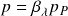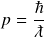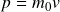# Momentum

The New Foundation Model replaces momentum in unit dimensions LMT-1 with inertial mass in unit dimension M to more accurately represent the physical phenomenon in formulas. Inertial mass can be translated into traditional units of momentum simply by multiplying the inertial mass by 𝑐.

To calculate the momentum of any particle–whether it has rest mass or not–one simply applies the wavelength operator to the Planck momentum potential.

## Standard formula

The standard momentum formula applies the wavelength operator to the Planck momentum potentialThe three unit dimensions of momentum quantify the particle’s wavelength as shown in the particle mechanics template:

Applying the wavelength operator to the maximum potential in each unit dimension produces two observable quantities–the particle’s wavelength and inertial mass. The time dimension is not an observable because velocity is not a physical representation of the particle’s momentum but a mathematical operator that quantifies its wavelength.

### Observable

The New Foundation Model uses the time variable 𝑇𝜆 in order to give the correct quantity of inertial mass. The ratio 𝑚/𝑇𝜆 is mathematically equivalent to 𝑚0/𝑇.

The standard form of the momentum formula uses the three equivalent forms of the wavelength operator multiplied by the Planck momentum.

## Expanded formula

The momentum formula also uses the expanded form of the wavelength operator to find momentum. The expanded wavelength operator is the product of the rest mass and momentum operators.

Substituting the expanded operators produces the same transformations in the traditional unit dimensions of momentum

The two operators produce the same observables from the Planck momentum potential

### Observable

The expanded form of the momentum formula applies the two operators to the Planck momentum potentialThe expanded form of the momentum formula uses three equivalent forms of the rest mass and momentum operators multiplied by the Planck momentum.

Because the momentum and velocity operators are equal, additional forms of the momentum formula can be created by substituting the velocity operator for the momentum operatorThis is the familiar form of the equation that produces the right answer but gives the wrong physical description of the particle or system. Applying these two operators to the maximum Planck potential gives the same observables.

### Observable

This alternate form of the expanded momentum formula uses three equivalent forms of the rest mass and velocity operators multiplied by the Planck momentum.

## Historical momentum formulas

The New Foundation Model momentum formula works for all types of elementary particles and systems. Different formulas have been used historically to calculate the momentum of particles with rest mass versus those without. The formula for photon momentumcan be converted to the New Foundation Model momentum formula by replacing Planck’s constant with fundamental units of length, mass, and time as shown in the following illustration

Photon momentum: conversion from historical formula to natural formula

The classical momentum formulacan also be converted to the New Foundation Model formula by showing the physical attributes of wavelength in place of the mathematically equivalent rest mass and velocity terms as shown in the following illustration

Momentum: conversion from historical formula to natural formula

Momentum